# Scientific Notation What is Scientific Notation Scientific notation

• Slides: 8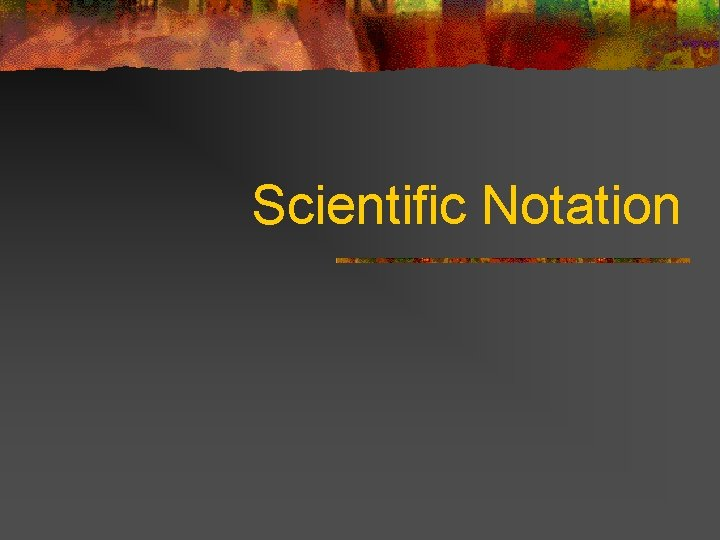Scientific Notation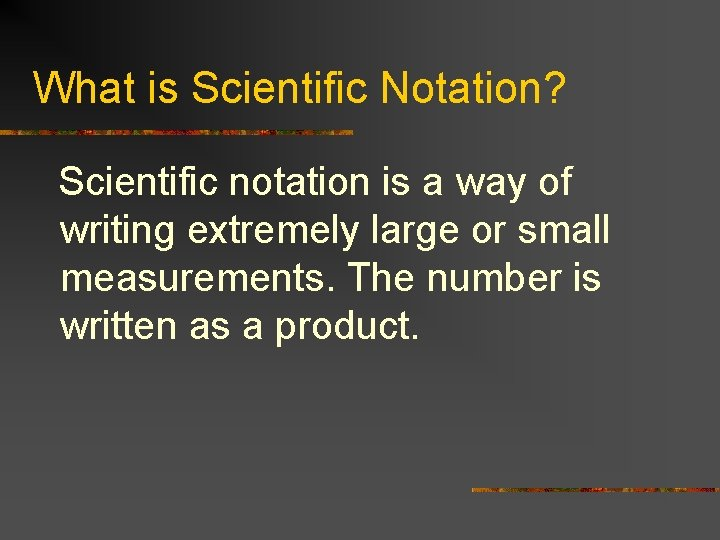What is Scientific Notation? Scientific notation is a way of writing extremely large or small measurements. The number is written as a product.What are the steps? 1. Write number 2. Write next factor as an exponent of such that it is 10 and count between 1 and decimal places 10. moved.Rules for Changing Exponents If you increase the If you decrease the exponent, increase the decrease the number (move decimal to the left) decimal to the right)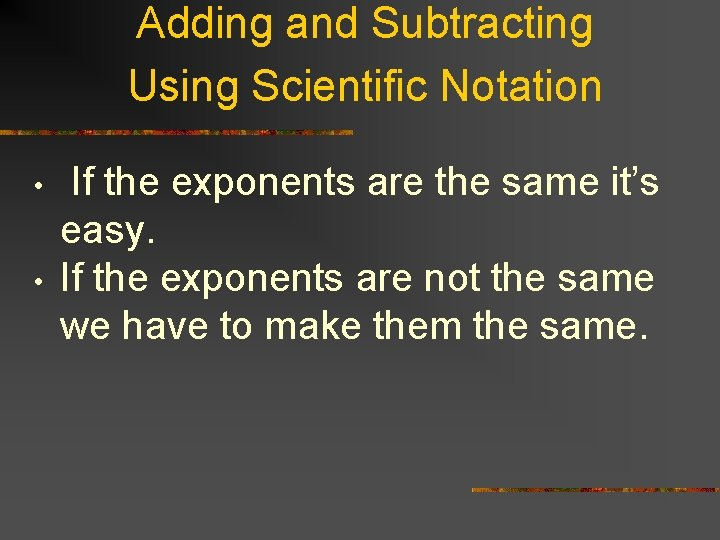Adding and Subtracting Using Scientific Notation • • If the exponents are the same it’s easy. If the exponents are not the same we have to make them the same.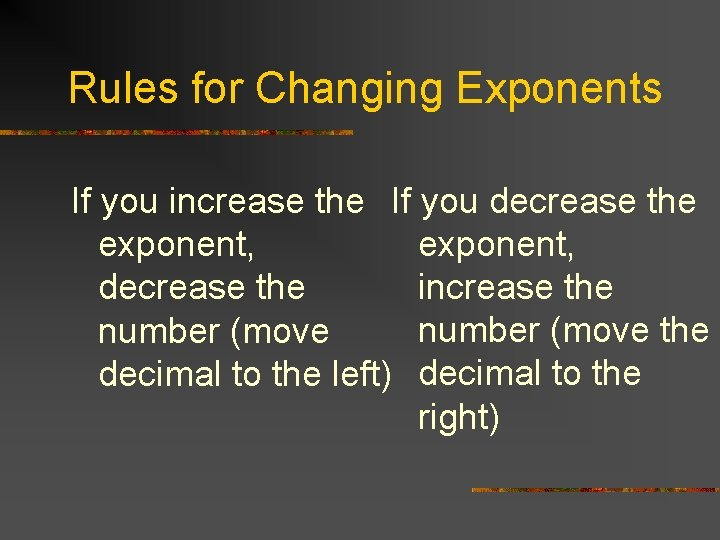Rules for Changing Exponents If you increase the If you decrease the exponent, increase the decrease the number (move decimal to the left) decimal to the right)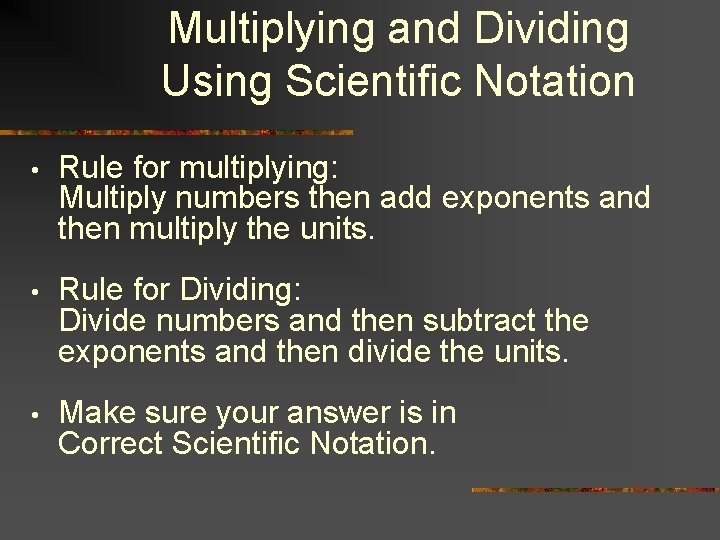Multiplying and Dividing Using Scientific Notation • Rule for multiplying: Multiply numbers then add exponents and then multiply the units. • Rule for Dividing: Divide numbers and then subtract the exponents and then divide the units. • Make sure your answer is in Correct Scientific Notation.Rules for Changing Exponents If you increase the If you decrease the exponent, increase the decrease the number (move decimal to the left) decimal to the right)